Processing ......FreeComputerBooks.com Links to Free Computer, Mathematics, Technical Books all over the World

Linear Algebra, Theory And Applications
Top Free Computer Networking Books 🌠 - 100% Free or Open Source!
• Title Linear Algebra, Theory And Applications
• Author(s) Kenneth Kuttler
• Publisher: Brigham Young University (December 10, 2022)
• Hardcover/Paperback N/A
• eBook PDF (466 pages)
• Language: English
• ISBN-10: N/A
• ISBN-13: N/A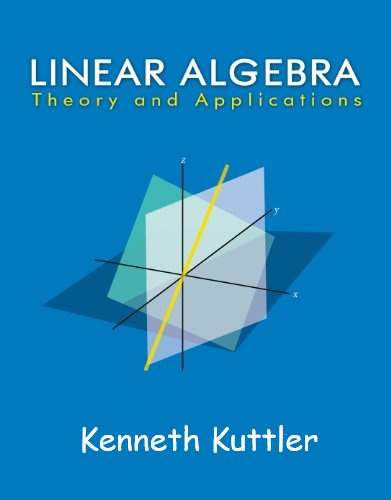Book Description

This is a book on linear algebra and matrix theory. While it is self contained, it will work best for those who have already had some exposure to linear algebra. It is also assumed that the reader has had calculus. Some optional topics require more analysis than this, however. I think that the subject of linear algebra is likely the most significant topic discussed in undergraduate mathematics courses. Part of the reason for this is its usefulness in unifying so many different topics.

This book gives a self- contained treatment of linear algebra with many of its most important applications. It is very unusual if not unique in being an elementary book which does not neglect arbitrary fields of scalars and the proofs of the theorems. It will be useful for beginning students and also as a reference for graduate students and others who need an easy to read explanation of the important theorems of this subject. It presents a self- contained treatment of the algebraic treatment of linear differential equation which includes all proofs. It also contains many different proofs of the Cayley Hamilton theorem. Other applications include difference equations and Markov processes, the latter topic receiving a more thorough treatment than usual, including the theory of absorbing states. In addition it contains a complete introduction to the singular value decomposition and related topics like least squares and the pseudo-inverse.

Most major topics receive more than one discussion, one in the text and others being outlined in the exercises. The book also gives directions for using maple in performing many of the difficult algorithms.

Linear algebra is essential in analysis, applied math, and even in theoretical mathematics. This is the point of view of this book, more than a presentation of linear algebra for its own sake. This is why there are numerous applications, some fairly unusual.

• N/A
Reviews, Ratings, and Recommendations: Related Book Categories: Read and Download Links:Similar Books:
•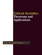Linear Algebra - Theorems and Applications (Hassan Abid Yasser)

This book contains selected topics in linear algebra, which represent the recent contributions in the most famous and widely problems. It includes a wide range of theorems and applications in different branches of linear algebra, etc.

•Applied Linear Algebra: Vectors, Matrices, and Least Squares

Combines straightforward explanations with a wealth of practical examples to offer an innovative approach to teaching linear algebra. Requiring no prior knowledge of the subject, it covers the aspects of linear algebra - vectors, matrices, and least squares

•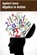Applied Linear Algebra in Action (Vasilios N. Katsikis)

This textbook contains a collection of six high-quality chapters. The techniques are illustrated by a wide sample of applications. This book is devoted to Linear Mathematics by presenting problems in Applied Linear Algebra of general or special interest.

•Linear Algebra (Jim Hefferon)

This textbook covers linear systems and Gauss' method, vector spaces, linear maps and matrices, determinants, and eigenvectors and eigenvalues. Each chapter has three or four discussions of additional topics and applications.

•Linear Algebra: A Course for Physicists and Engineers (Arak Mathai)

This textbook on linear algebra is written to be easy to digest by non-mathematicians. It introduces the concepts of vector spaces and mappings between them without too much theorems and proofs. Various applications of the formal theory are discussed as well.

•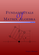Fundamentals of Matrix Algebra (Gregory Hartman)

A college (or advanced high school) level text dealing with the basic principles of matrix and linear algebra. It covers solving systems of linear equations, matrix arithmetic, the determinant, eigenvalues, and linear transformations.

•Matrix Algebra (Marco Taboga)

This is a course in matrix algebra, with a focus on concepts that are often used in probability and statistics. Matrix algebra is one of the most important areas of mathematics for data analysis and for statistical theory.

•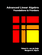Advanced Linear Algebra: Foundations to Frontiers (Robert Geijn)

The focus is on numerical linear algebra, the study of how theory, algorithms, and computer arithmetic interact. These materials keep the learner engaged by intertwining text, videos, exercises, and programming activities in consumable chunks.

•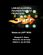Linear Algebra: Foundations to Frontiers (M. Myers, et al.)

This book is an introduction to the basic concepts of linear algebra, along with an introduction to the techniques of formal mathematics. It begins with systems of equations and matrix algebra before moving into the advanced topics.

•Computational Linear Algebra and N-dimensional Geometry

This undergraduate textbook on Linear Algebra and n-Dimensional Geometry, in a self-teaching style, is invaluable for sophomore level undergraduates in mathematics, engineering, business, and the sciences.

•A First Course in Linear Algebra (Robert A Beezer)

Linear Algebra: Foundations to Frontiers (LAFF) is packed full of challenging, rewarding material that is essential for mathematicians, engineers, scientists, and anyone working with large datasets.

•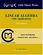Linear Algebra with Applications (W. Keith Nicholson)

The aim of the text is to achieve a balance among computational skills, theory, and applications of linear algebra. It is a relatively advanced introduction to the ideas and techniques of linear algebra targeted for science and engineering students.

Book Categories
 :All CategoriesTop Free BooksRecent BooksMiscellaneous BooksComputer EngineeringComputer LanguagesComputer ScienceData Science/DatabasesJava and Java EE (J2EE)Linux and UnixMathematicsMicrosoft and .NETMobile ComputingNetworking and CommunicationsSoftware EngineeringSpecial TopicsWeb Programming
Other Categories